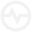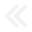Zeroing is a timed arithmetic puzzle game. You are given five to eight numbers placed in an arithmetic sequence on the nine squared game board. You must solve this sequence before time expires. The arithmetic sequence must result in zero to win the game.

Solving PuzzlesTo solve puzzles, you combine two numbers in the puzzle, either horizontally or vertically. Continue to combine numbers until there are no more numbers to combine. The resulting number from the combination depends on how you combine them.

Combining Small to BigIf you combine a small number to a big number, the small number is added to the big number.
Example: Combining 3 to 4 results in 7.

Combining Big to SmallOn the other hand, if you combine a big number to a small number, the small number is subtracted from the big number.
Example: Combining 8 to 7 results in 1.

Combining Similar NumbersAnd when you combine the same numbers, it will subtract the numbers.
Example: Combining 5 to 5 results in 0.

You continue to combine numbers until you clean the board and win the game.

If you get stuck with a tricky puzzle, you can use the game’sLifeline options to help you figure out the solution. These options include;

1.Additional 2 undo moves
2.Additional 30 seconds to solve the puzzle and
3.Reveal first 2 moves (can only be used if puzzle pieces are complete)

Just remember that using a lifeline decreases your victory score so try to solve the puzzle as fast and as perfect as you can!

This is Zeroing; a game that will challenge your math and memory skills and hone them with endless puzzles.